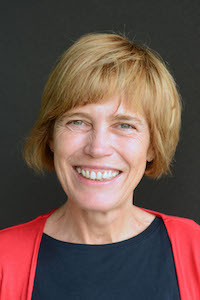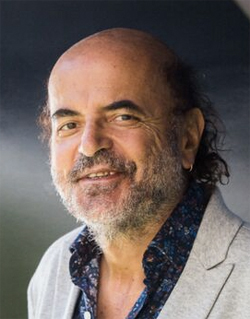43rd International Symposium on Mathematical Foundations of Computer Science August 27-31, 2018, Liverpool (UK)MFCS 2018 is organized in coopperation with EATCS

Important Dates
Friday, 20th April, 2018
Tuesday, 24th April, 2018
Tuesday, 12th June, 2018
Monday, 25th June, 2018
Friday, 20th July, 2018
Conference dates:
27th-31st August, 2018

# Invited Speakers

### Christel Baier(TU Dresden, Germany)

On energy conditions and stochastic shortest path problems for Markov Decision Processes
Markov decision processes (MDP) are widely used to formalize algorithmic problems where the task is to find a policy for traversing a weighted probabilistic graph structure in a somehow optimal way. Examples are the stochastic shortest-path problem where the goal is to minimize the expected accumulated weight until reaching a target or decision problems for MDPs with energy constraints that, e.g., aims to ensure that almost surely a target will be reached while the accumulated weight (``energy'') meets a given bound.
The talk will discuss solutions for such and related problems for MDPs with integer weights. These rely on a new classification of so-called end components of MDPs according to their limiting behavior with respect to the accumulated weights. This classification will be used to show that the stochastic shortest path problem is solvable in polynomial time for arbitrary finite-state MDPs, generalizing previous results for sub-classes of MDPs. Furthermore, it will be used to provide algorithms for deciding the existence of a policy ensuring that a weight-bounded (repeated) reachability condition or holds almost surely or with positive probability, and the analogous problems for universal rather than existential quantification over policies.

### Olivier Bournez(LIX, France)

Descriptive Mathematics and Computer Science with Polynomial Ordinary Differential Equations.
We will see that many continuous and discrete concepts from mathematics and computer science can be presented using ordinary differential equations. Basically, we will start from the following observation: if you know what 0, 1, -1 are, as well as what an addition and a multiplication are, and if you remember what an ordinary differential equation is, then you can define and program many concepts from Mathematics and Computer Science. In particular we will present/rediscover descriptive complexity, computability and complexity using polynomial ordinary differential equations only. A title for this talk could also be "Programming with Ordinary Differential Equations”, as these questions also relate to analog models of computations, and in particular to the 1941 General Purpose Analog Computer of Claude Shannon. In some way, we are rediscovering the forgotten art of their programming, and we are only starting to understand the true power of these very old models.

### Herbert Edelsbrunner(IST, Austria)

Tri-partition of a simplicial complex
We prove that for every simplicial complex, K, and every dimension, p, there is a partition of the p-simplices into a maximal p-tree, a maximal p-cotree, and the remaining p-simplices defining the p-th homology of K. Given a monotonic order of the simplices, this tri-partition is unique and can be computed by matrix reduction. Collecting the sets over all monotonic orders, we get matroids over the set of p-simplices.
(Joint work with Katharina Oelsboeck)

### Leslie Ann Goldberg(University of Oxford, UK)

Computational Complexity and the Independence Polynomial
The independence polynomial is one of the most well-studied graph polynomials, arising in combinatorics and computer science. It is also known in statistical physics as the "partition function of the hard-core model". After describing the polynomial, I will tell you something about the complexity of approximating this polynomial, including the now-classical breakthrough results of Weitz and Sly, incursions into the complex plane by Harvey, Srivastava, and Vondr\'ak and by Patel and Regts and finally more recent work using tools from complex analysis by Peters and Regts and also in joint work with Bezakova, Galanis, and Stefankovic.